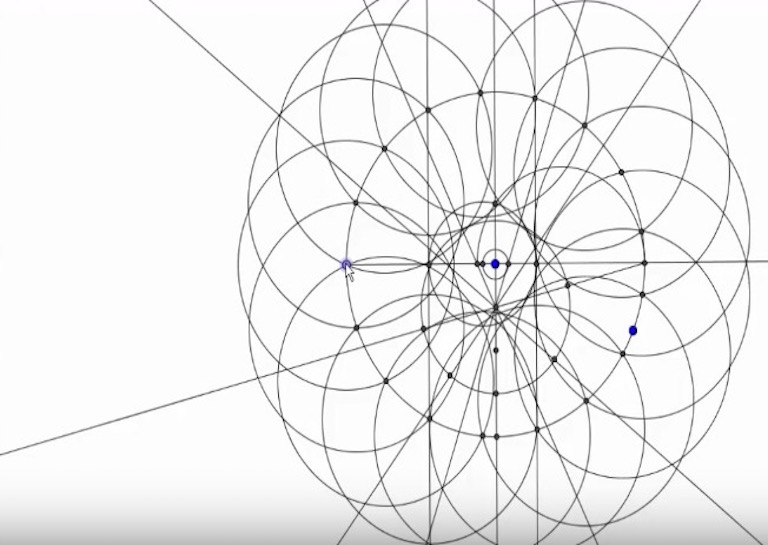# How to Create a Regular Heptadecagon using Math!

•A regular heptadecagon, or 17 sided polygon, was known to have existed by mathematicians for many years, but creating one proved to be a greater challenge. A regular Heptadecagon is one where every angle has the same number of degrees and each side has the same length. It is near impossible to create by simply drawing, but it can be done through a series of movements using a compass and straight edge. In order to understand how difficult a task this is, watch the video below from the Maths Doctor which will take you through all of the steps.

17 is a Fermat prime, which means that creating a 17 sided polygon is possible using only a compass. A regular heptadecagon was first created by 19-year-old Carl Friedrich Gauss in 1796 in a groundbreaking proof. The proof in regards to this 17-gon's construction marked the first major breakthrough in polygon construction in over 2,000 years.

Apart from a heptadecagon, there are also heptadecagrams, which are 17 sided star polygons. There are multiple ways to create actual heptadecagrams ranging from ones with short sides to ones that stretch nearly the entire radius of a representative circle.[Image Source: Maths Doctor]

The proof that the Maths Doctor used to construct the heptadecagon was first created by Herbert William Richmond in 1893. The process can be done on paper using a compass and straight edge, aside from being done in computer programs. There are many other ways to construct a heptadecagon besides the one shown, which can be seen here.

Most Popular

## SEE ALSO: Turn a Cross into a Square in Two Cuts – Can You do It?SHOW COMMENT (1)